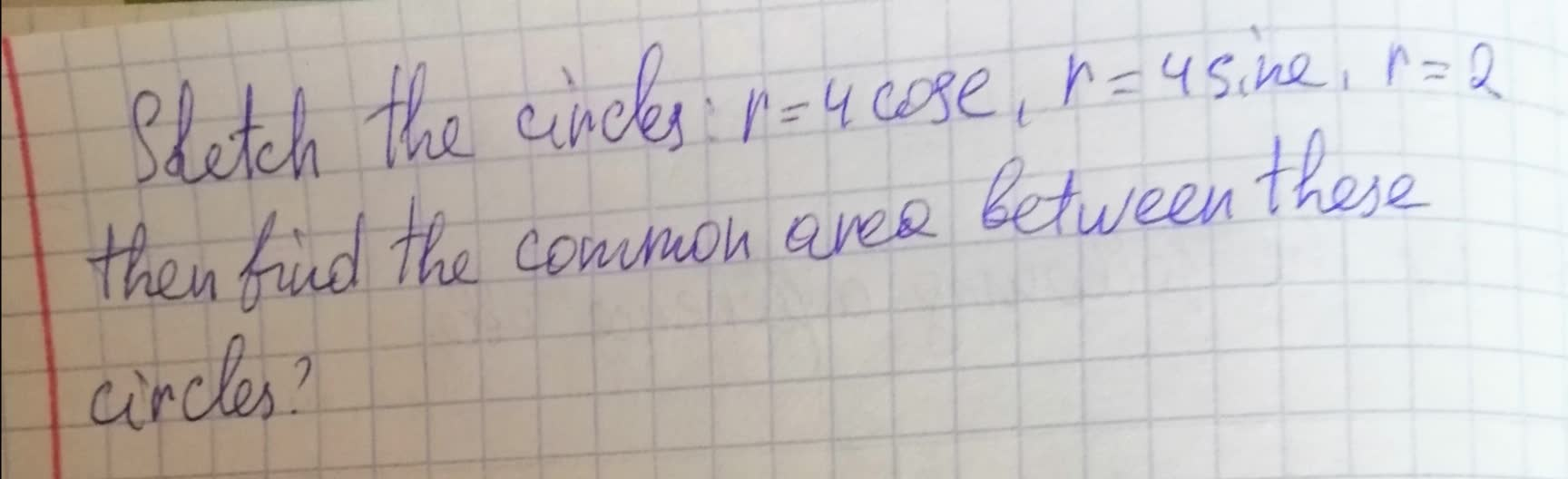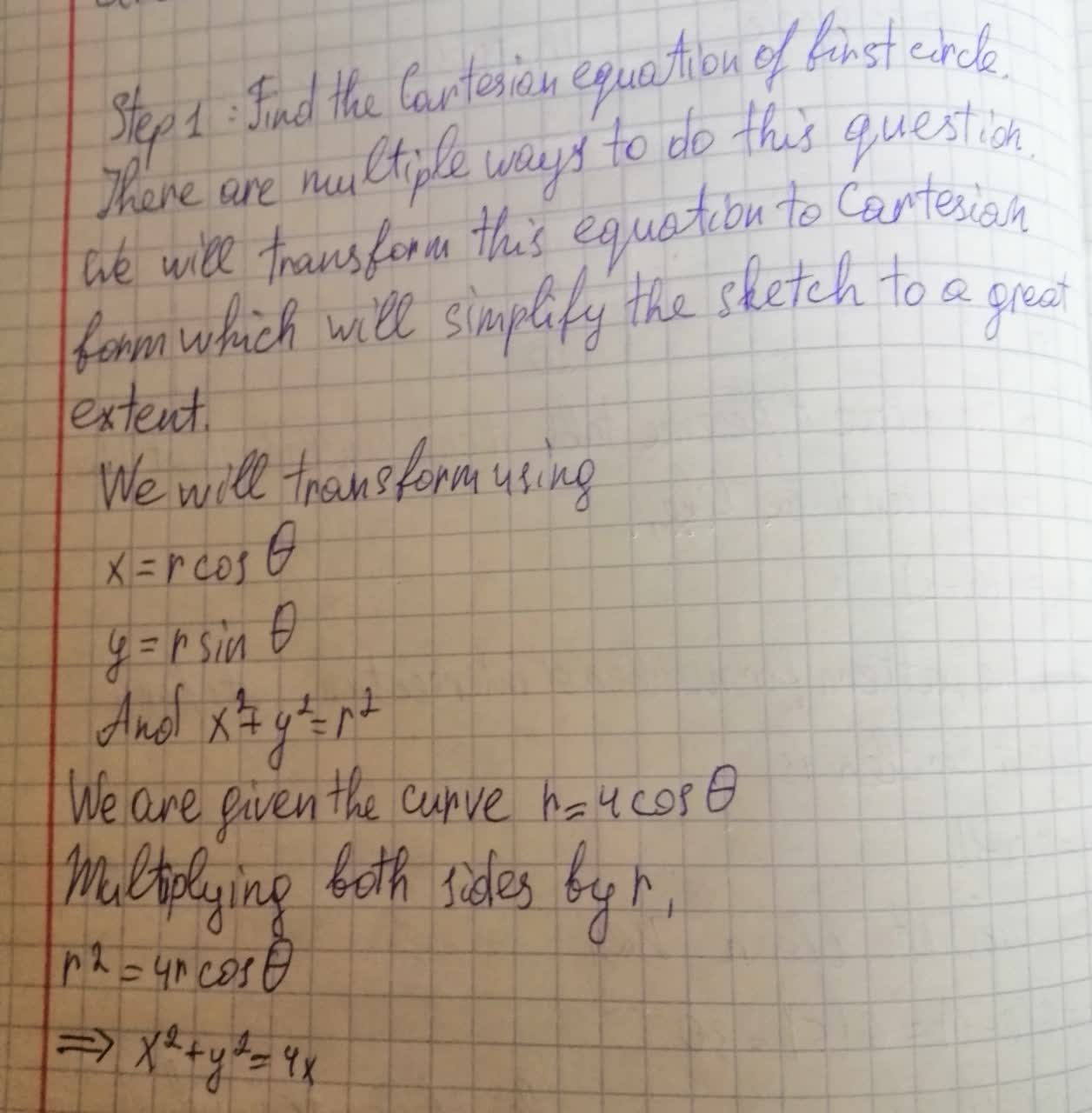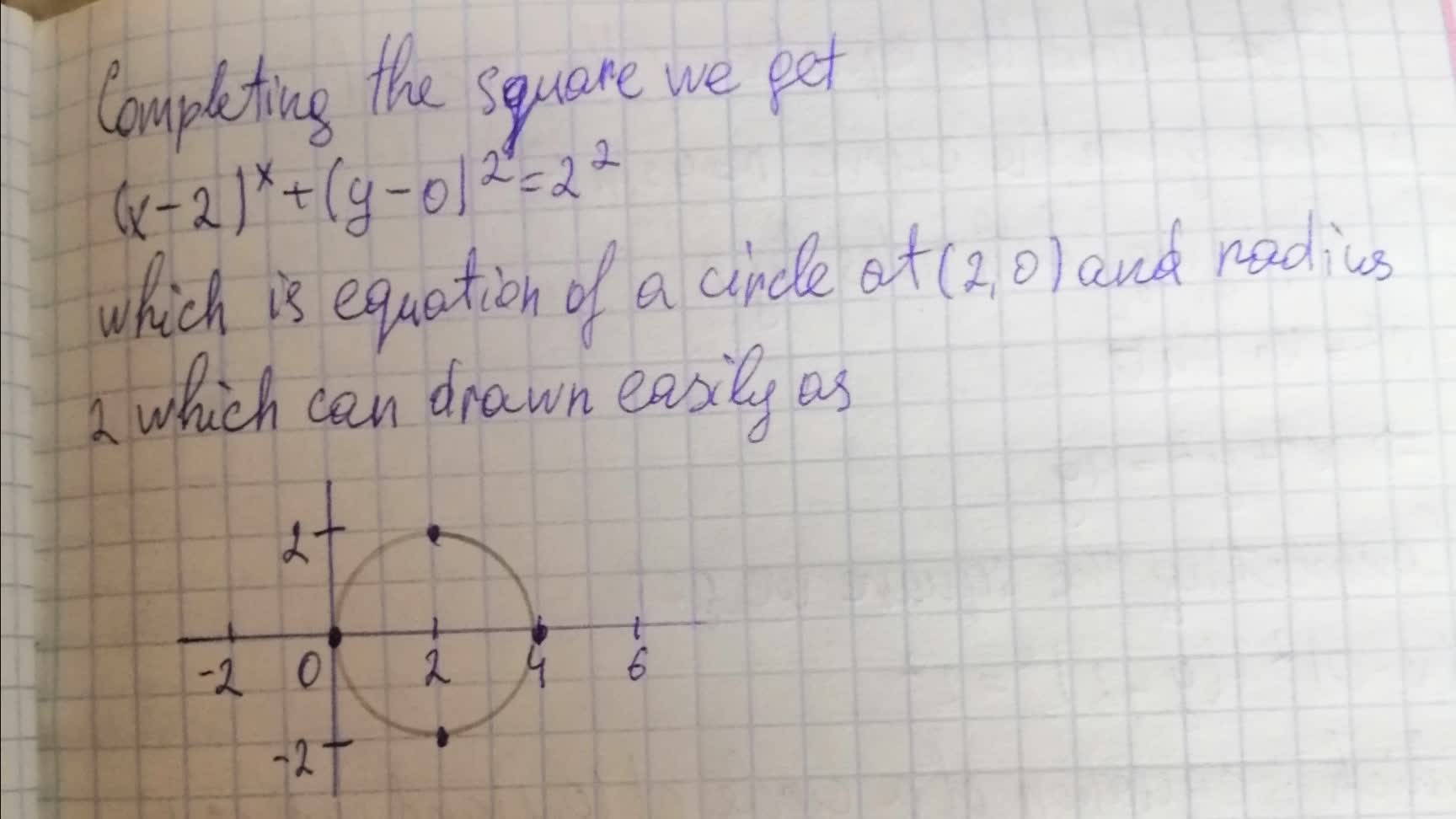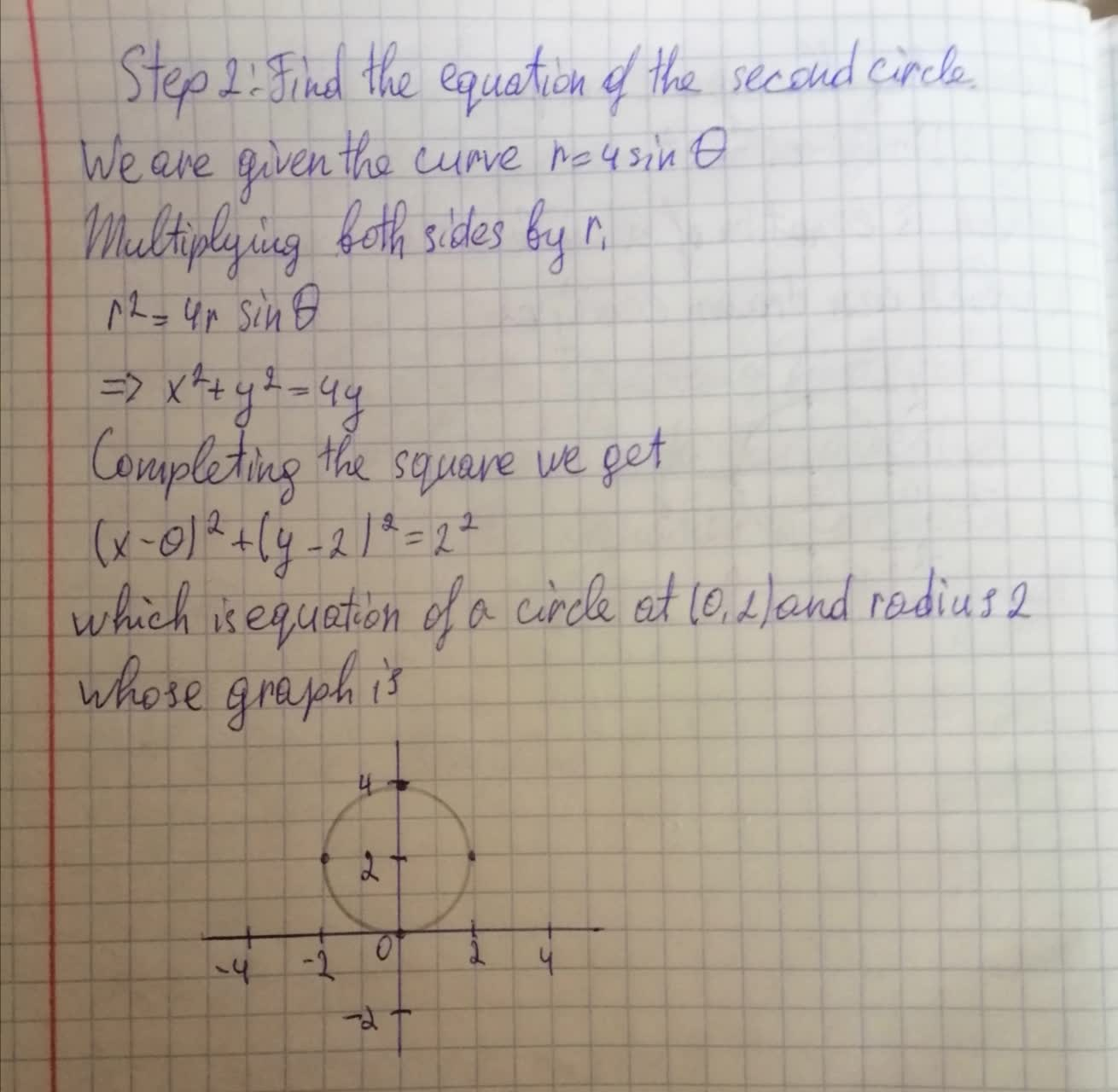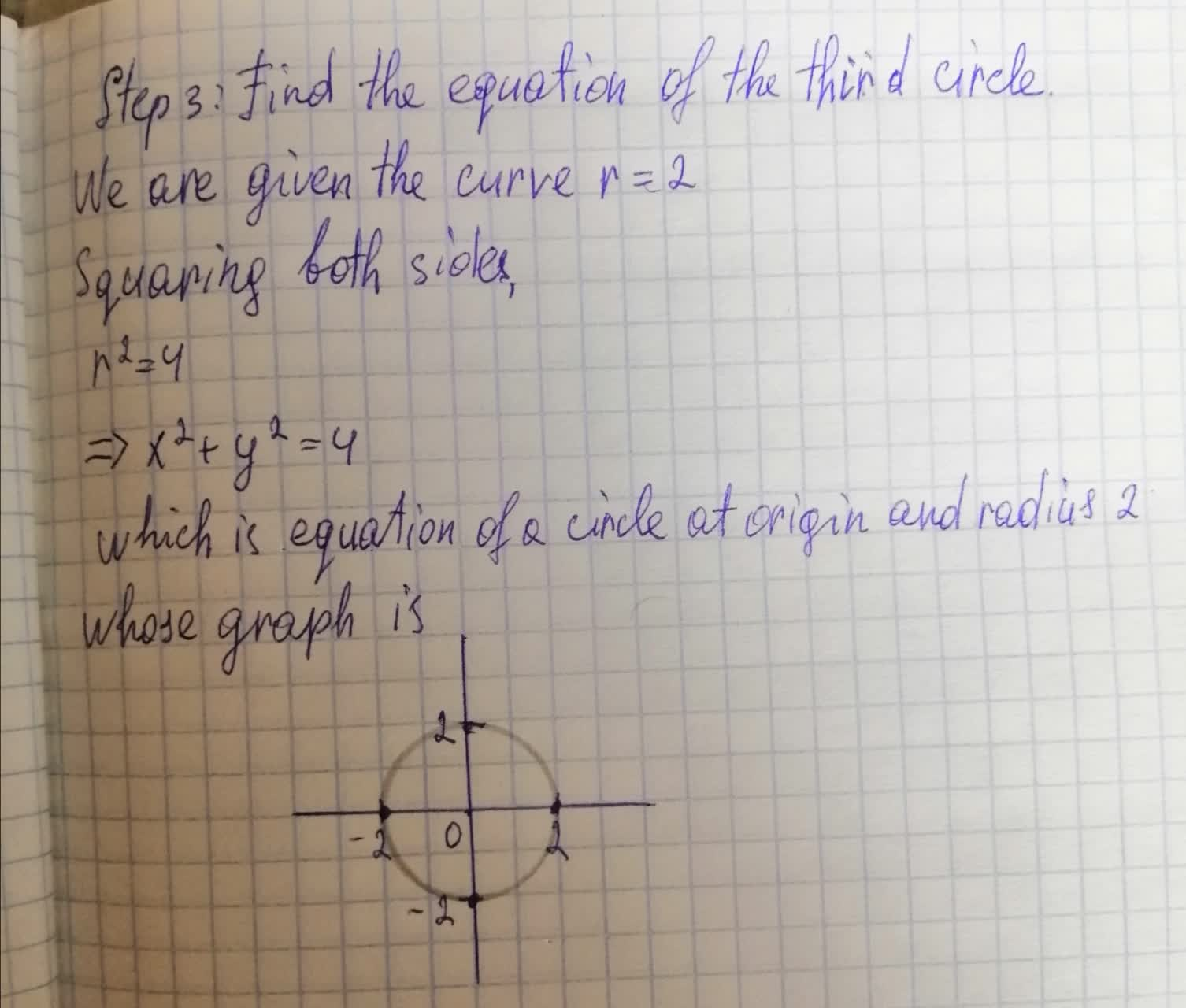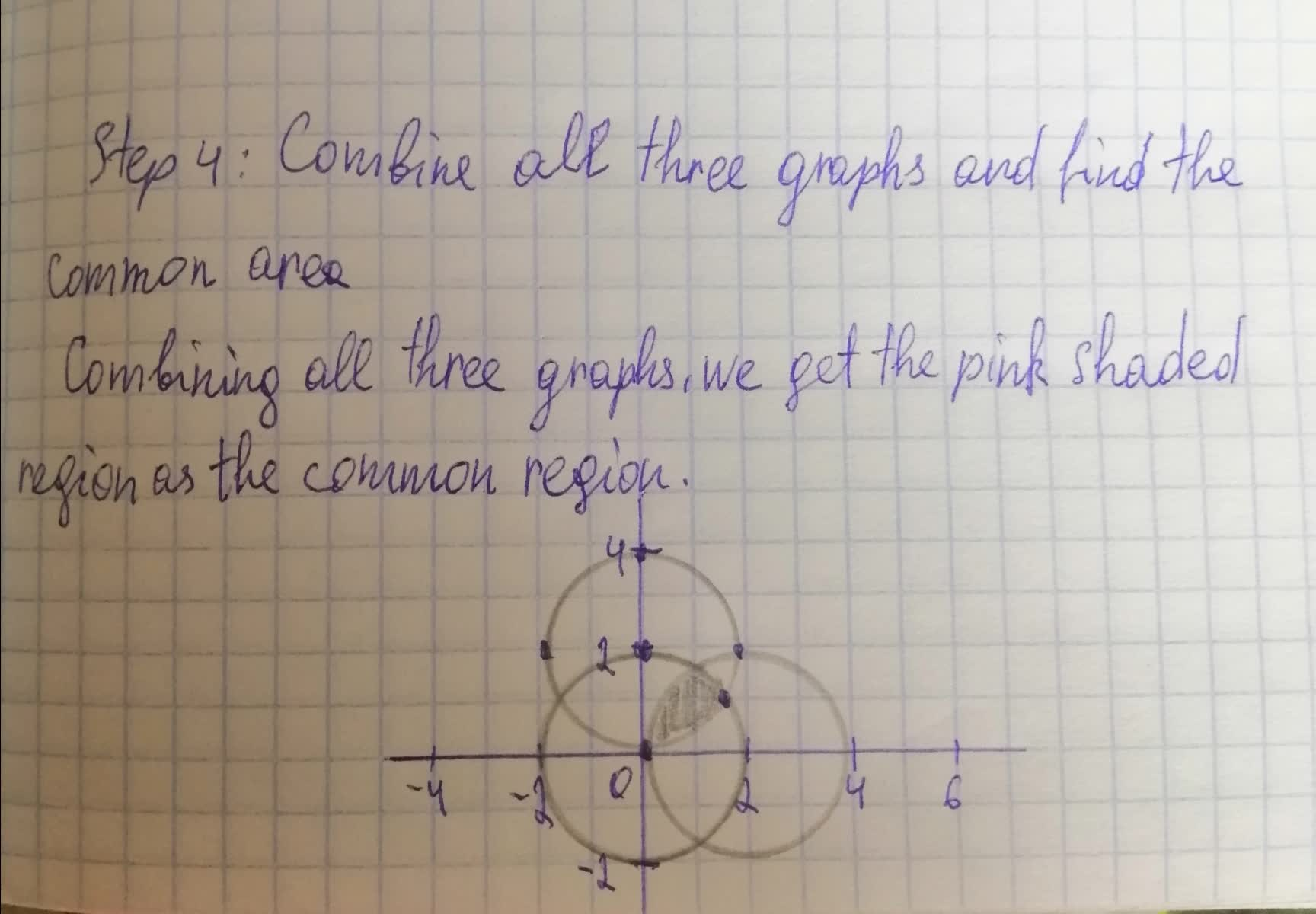Question# Sketch the circles: r = 4cose, r = 4sine, and r = 2 then find the common area between these circles?

Circles
ANSWEREDSketch the circles: r = 4cose, r = 4sine, and r = 2 then find the common area between these circles?